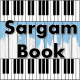# Dance Monkey Sargam Notes Tones and I

Dance Monkey Sargam Notes Tones and I Available On Sargam Book. Dance Monkey Easy Harmonium Notes For Beginners. Dance Monkey Harmonium Notes On Sargam Book.

Song : Dance Monkey
Singer : Tones and I
Classical Sargam Notes : www.sargambook.com
Guitar / Piano Chords : https://chords.pianodaddy.com

They say, “Oh my God, I see the way you shine
Ma(t) Ga, Ma(t) Ga, Ma(t) Ga, Ma(t) Ga, Ma(t) Dha Dha(k)
Take your hands, my dear, and place them both in mine”
Dha Dha Dha(k), Ma(t) Ma(t) Ga Ma(t), Ga Ma(t) Dha Dha(k)
You know you stopped me dead while I was passing by
Ga Ma(t) Ga, Ma(t) Ga Ma(t), Ga Ma(t) Ga Ma(t) Dha Dha(k)
And now I beg to see you dance just one more time
Ma(t) Dha Dha Dha(k), Ga Ma(t) Ga Ma(t), Ga Ma(t) Dha Dha(k)

Ooh, I see you, see you, see you every time
RE(k), Ni Dha Ni Dha Ni Dha, Ni Dha Dha(k) Ma(t) Ga
And oh my, I, I like your style
Ma(t) Dha Dha Dha(k), Ma(t) Ga Ma(t) Ga Ma(t) Ma(t), Dha Dha(k)
You, you make me, make me, make me wanna cry
Ni.. Dha(k) Ma(t), Dha(k) Ma(t), Dha(k) Ma(t), Ma(t) Ma(t) Dha
And now I beg to see you dance just one more time
Ma(t) Dha Dha Dha(k), Ga Ma(t) Ga Ma(t) Ga, Ma(t) Dha Dha(k)

So they say
Ma(t) Ma(t) Ma(t)
Dance for me, dance for me, dance for me, oh-oh-oh
Dha Dha Dha, Dha(k) Dha(k) Dha(k), Ma(t) Ma(t) Ma(t)
Dha(k) Ma(t) Dha Dha(k) Ma(t)
I’ve never seen anybody do the things you do before
Re(k) Dha Dha Dha Dha, Dha(k) Dha(k) Dha(k) Dha(k)
Ma(t) Ma(t) Ma(t) Ma(t), Dha(k) Ma(t), Dha Dha(k) Ma(t)
They say
Ma(t) Ma(t), Ma(t)

Move for me, move for me, move for me, ayy-ayy-ayy
Dha Dha Dha, Dha(k) Dha(k) Dha(k), Ma(t) Ma(t) Ma(t)
Dha(k) Ma(t) Dha Dha(k) Ma(t)
And when you’re done, I’ll make you do it all again
Re(k) Dha Dha Dha Dha, Dha(k) Dha(k) Dha(k) Dha(k)
Ma(t) Ma(t) Ma(t) Ma(t), Dha(k) Ma(t), Dha Dha(k) Ma(t)

These are demo notes for respective song. You can try it on your instrument. If it works for you and you are comfortable to play along with our notes, you can simply get full notes by paying us. Just click the Buy Now button below and see our packages.#### Titiksha

Titiksha Telang is an Indian Musician & Singer. She is MA in Indian Classical Music & Owner of Sargam Book Website.